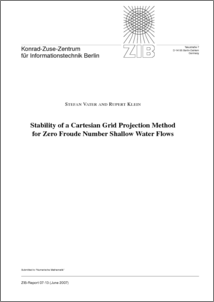Repository: Freie Universität Berlin, Math Department

# Stability of a Cartesian grid projection method for zero Froude number shallow water flows

Vater, S. and Klein, R. (2007) Stability of a Cartesian grid projection method for zero Froude number shallow water flows. Technical Report. Konrad-Zuse-Zentrum für Informationstechnik Berlin (ZIB).Preview
PDF - Published Version
669kB

## Abstract

In this paper a Godunov-type projection method for computing approximate solutions of the zero Froude number (incompressible) shallow water equations is presented. It is second-order accurate and locally conserves height (mass) and momentum. To enforce the underlying divergence constraint on the velocity field, the predicted numerical fluxes, computed with a standard second order method for hyperbolic conservation laws, are corrected in two steps. First, a MAC-type projection adjusts the advective velocity divergence. In a second projection step, additional momentum flux corrections are computed to obtain new time level cell-centered velocities, which satisfy another discrete version of the divergence constraint. The scheme features an exact and stable second projection. It is obtained by a Petrov-Galerkin finite element ansatz with piecewise bilinear trial functions for the unknown incompressible height and piecewise constant test functions. The stability of the projection is proved using the theory of generalized mixed finite elements, which goes back to Nicolaïdes (1982). In order to do so, the validity of three different inf-sup conditions has to be shown. Since the zero Froude number shallow water equations have the same mathematical structure as the incompressible Euler equations of isentropic gas dynamics, the method can be easily transfered to the computation of incompressible variable density flow problems.

Item Type: Monograph (Technical Report) Finite Volumes, Projection Methods, Shallow Water Equations Mathematical and Computer Sciences > Mathematics > Numerical Analysis Department of Mathematics and Computer Science > Institute of Mathematics > Geophysical Fluid Dynamics Group 538 Ulrike Eickers 13 Jul 2009 12:52 03 Mar 2017 14:40

Repository Staff Only: item control page